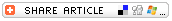## Search formBack to Math Fun (Volume 3) Lesson Plan

# Decimal BingoSubjects

• Mathematics
--Arithmetic

• 3-5
• 6-8
• 9-12

Brief Description

This BINGO game reinforces computation with decimals, but the lesson can be used to reinforce almost any math skill.

Objectives

Students will

• solve math problems correctly and mark appropriate squares on a BINGO card they have prepared.

Keywords

math, BINGO, game, decimal

• Math BINGO card
• Twenty-four (24) math-problem "flash cards containing one math problem per card. Each card should be large enough that students can see and copy the problem when you hold up the card.

Lesson Plan

This lesson can be used to reinforce most any math skill. Simply adapt the instructions below for a skill you are teaching or a skill your students have mastered.

Before the Lesson
Before the lesson, create at least 24 math problems for students to solve. Write each problem on a card large enough for students to see and copy the problem when you hold it up.

The Lesson
Provide each student with a copy of the Math BINGO card. Write on a chalkboard or chart the solutions to the 24 problems you have created for this game. Have students randomly copy each solution into one of the squares on their BINGO cards.

Emphasize that the solutions should be copied with care. If students copy a solution incorrectly, they will not be able to find that number on their cards.

If you teach younger students, you might opt to have students fold a sheet of math paper to create a BINGO card with only 16 squares.

One at a time, display the math-problem "flash cards" you have prepared. Give students a set amount of time to solve the problem on that card. (The time limit should provide enough time for almost all of the students to solve the problem.) When students have solved the problem, they must find the solution on their BINGO card and put an X through it. Continue showing flash cards until one student has five Xs in a row. The first student to have five Xs calls out "BINGO!" and is the winner of the game.

Homework BINGO
This activity can be used as an alternative homework assignment. Have students prepare the BINGO card as above before handing out the assignment. Then give them a sheet of 24 math problems for homework. They must solve the problems in the sequence they appear on the homework sheet. As they complete each problem, they mark an X on that solution on the BINGO card. They only have to complete as many of the homework problems as it takes for them to get a BINGO. In other words, if they get a BINGO after solving only 10 problems, they do not need to complete problems 11 to 24.

Assessment

Use the Homework BINGO assignment immediately above as an assessment activity.

Lesson Plan Source

Education World

Submitted By

Gary Hopkins

National Standards

MATHEMATICS: Number and Operations